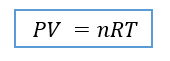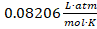# Problem: Chlorine is widely used to purify municipal water supplies and to treat swimming pool water. Suppose that the volume of a particular sample of Cl2 gas is 8.70 L at 895 torr and 24 oC.How many grams of Cl2 are in the sample?

###### FREE Expert Solution

Recall the ideal gas law equation:Where:

P , pressure = 895 torr

n , number of moles, unknown

V , volume = 8.7 L

R, gas constant =** Values for the gas constant can be found online or in textbooks

T, temperature in K, but given: T = 24°C

To solve for grams of Cl2, we need to do these steps:

1. Convert:
• Temperature from °C to K
• Pressure from torr to atm
2. Solve for the molar mass of Cl2
3. Solve for n, moles of Clusing Ideal Gas Law
4. Do a mole to mass calculation
79% (314 ratings)###### Problem Details

Chlorine is widely used to purify municipal water supplies and to treat swimming pool water. Suppose that the volume of a particular sample of Cl2 gas is 8.70 L at 895 torr and 24 oC.

How many grams of Cl2 are in the sample?